Need help? Email help@shout.education

# Copper

This page looks at some aspects of copper chemistry required for UK A-level exams. The chemistry of copper(II) is mainly summarised from elsewhere on the site, with links available to more detailed explanations. The page also covers some simple copper(I) chemistry.

## Reactions of Copper(II) Ions in Solution

The simplest ion that copper forms in solution is the typical blue hexaaquacopper(II) ion – [Cu(H2O)6]2+.

Note: If you aren't happy about complex ions (including the way they are bonded and named), it would pay you to follow this link and explore the first couple of pages in the complex ions menu before you go on.

### The Reaction of Hexaaquacopper(II) Ions With Hydroxide Ions

Hydroxide ions (from, say, sodium hydroxide solution) remove hydrogen ions from the water ligands attached to the copper ion.

Once a hydrogen ion has been removed from two of the water molecules, you are left with a complex with no charge – a neutral complex. This is insoluble in water and a precipitate is formed.

[\text{Cu}(\text{H}_2\text{O})_6]^{2+} + \color{#467abf}{2\text{OH}^-} \longrightarrow [\text{Cu}(\text{H}_2\text{O})_4(\text{OH})_2] + \color{#467abf}{2\text{H}_2\text{O}}

Note: The colour coding is to show that this isn't a ligand exchange reaction. The oxygens which were originally attached to the copper are still attached in the neutral complex.

In the test-tube, the colour change is: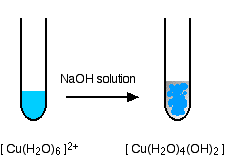Note: You will find the reactions between hexaaqua ions and hydroxide ions discussed in detail if you follow this link.

### Reactions of Hexaaquacopper(II) Ions With Ammonia Solution

The ammonia acts as both a base and a ligand. With a small amount of ammonia, hydrogen ions are pulled off the hexaaqua ion exactly as in the hydroxide ion case to give the same neutral complex.

[\text{Cu}(\text{H}_2\text{O})_6]^{2+} + 2\text{NH}_3 \longrightarrow [\text{Cu}(\text{H}_2\text{O})_4(\text{OH})_2] + 2{\text{NH}_4}^+

That precipitate dissolves if you add an excess of ammonia.

The ammonia replaces water as a ligand to give tetraamminediaquacopper(II) ions. Notice that only 4 of the 6 water molecules are replaced.

[\text{Cu}(\text{H}_2\text{O})_6]^{2+} + 4\text{NH}_3 \longrightarrow [Cu(\text{NH}_3)_4(\text{H}_2\text{O})_2]^{2+} + 4\text{H}_2\text{O}

Note: You might wonder why this second equation is given starting from the original hexaaqua ion rather than the neutral complex. Explaining why the precipitate redissolves is quite complicated. You will find the explanation in full on the page about the reactions between hexaaqua ions and ammonia solution.

The colour changes are: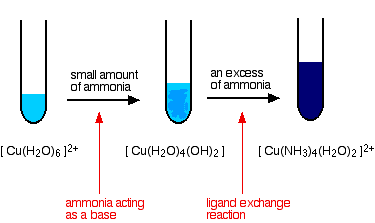### The Reaction of Hexaaquacopper(II) Ions With Carbonate Ions

You simply get a precipitate of what you can think of as copper(II) carbonate.

\text{Cu}^{2+}_{(aq)} + {\text{CO}_3}^{2-}_{(aq)} \longrightarrow \text{CuCO}_{3(s)}Note: Only one of the UK A-level Exam Boards wants this, and this is the simplification that they make. In fact, the precipitate is better described as a basic carbonate with a formula of the type xCuCO3,yCu(OH)2,zH2O.

You will find the reactions between hexaaqua ions and carbonate ions discussed in detail if you follow this link.

### A Ligand Exchange Reaction Involving Chloride Ions

If you add concentrated hydrochloric acid to a solution containing hexaaquacopper(II) ions, the six water molecules are replaced by four chloride ions.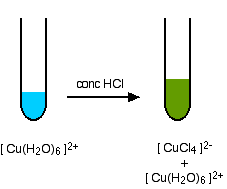The reaction taking place is reversible.

[\text{Cu}(\text{H}_2\text{O})_6]^{2+} + 4\text{Cl}^- \xrightleftharpoons{} [\text{CuCl}_4]^{2-} + 6\text{H}_2\text{O}

Because the reaction is reversible, you get a mixture of colours due to both of the complex ions.

You may find the colour of the tetrachlorocuprate(II) ion variously described as olive-green or yellow. If you add water to the green solution, it returns to the blue colour.

Note: You will find this reaction explored in more detail on a page about ligand exchange reactions.

### The Reaction of Hexaaquacopper(II) Ions With Iodide Ions

#### The simple reaction

Copper(II) ions oxidise iodide ions to iodine, and in the process are themselves reduced to copper(I) iodide.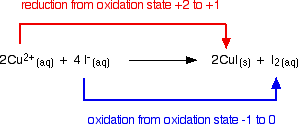Note: If you need to, you can find out more about oxidation states by following this link.

The initial mucky brown mixture separates into an off-white precipitate of copper(I) iodide under an iodine solution.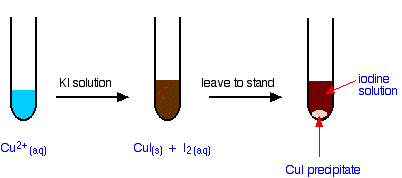Note: I've had several attempts at getting this colour right, but my software keeps changing it slightly as I adapt the diagram for Web use. The colour of the iodine solution actually varies quite a lot depending on its concentration, so I'm not too bothered about this!

#### Using this reaction to find the concentration of copper(II) ions in solution

If you pipette a known volume of a solution containing copper(II) ions into a flask, and then add an excess of potassium iodide solution, you get the reaction we have just described.

2\text{Cu}^{2+}_{(aq)} + 4\text{I}^-_{(aq)} \longrightarrow 2\text{CuI}_{(s)} + \text{I}_{2(aq)}

You can find the amount of iodine liberated by titration with sodium thiosulfate solution.

{2\text{S}_2\text{O}_3}^{2-}_{(aq)} + \text{I}_{2(aq)} \longrightarrow {\text{S}_4\text{O}_6}^{2-}_{(aq)} + 2\text{I}^-_{(aq)}

As the sodium thiosulfate solution is run in from a burette, the colour of the iodine fades. When it is almost all gone, you add some starch solution. This reacts reversibly with iodine to give a deep blue starch-iodine complex which is much easier to see.

You add the last few drops of the sodium thiosulfate solution slowly until the blue colour disappears.

If you trace the reacting proportions through the two equations, you will find that for every 2 moles of copper(II) ions you had to start with, you need 2 moles of sodium thiosulfate solution.

If you know the concentration of the sodium thiosulfate solution, it is easy to calculate the concentration of the copper(II) ions.

Note: If you aren't very good at titration calculations, you might be interested in my chemistry calculations book. This particular example is worked through in detail on pages 66-67.

## Some Essential Copper(I) Chemistry

### The Disproportionation of Copper(I) Ions in Solution

Copper(I) chemistry is limited by a reaction which occurs involving simple copper(I) ions in solution. This is a good example of disproportionation – a reaction in which something oxidises and reduces itself.

Copper(I) ions in solution disproportionate to give copper(II) ions and a precipitate of copper.

##### The reaction is:Any attempt to produce a simple copper(I) compound in solution results in this happening.

For example, if you react copper(I) oxide with hot dilute sulfuric acid, you might expect to get a solution of copper(I) sulfate and water produced. In fact you get a brown precipitate of copper and a blue solution of copper(II) sulfate because of the disproportionation reaction.

\text{Cu}_2\text{O}_{(s)} + \text{H}_2\text{SO}_{4(conc.)} \longrightarrow \text{Cu}{(s)} + \text{CuSO}_{4(aq)} + \text{H}_2\text{O}_{(l)}

### Stabalising the Copper(I) Oxidation State

#### Insoluble copper(I) compounds

We've already seen that copper(I) iodide is produced as an off-white precipitate if you add potassium iodide solution to a solution containing copper(II) ions. The copper(I) iodide is virtually insoluble in water, and so the disproportionation reaction doesn't happen.

Similarly copper(I) chloride can be produced as a white precipitate (reaction described below). Provided this is separated from the solution and dried as quickly as possible, it remains white. In contact with water, though, it slowly turns blue as copper(II) ions are formed.

The disproportionation reaction only occurs with simple copper(I) ions in solution.

#### Copper(I) complexes

Forming copper(I) complexes (other than the one with water as a ligand) also stabalises the copper(I) oxidation state.

For example, both [Cu(NH3)2]+ and [CuCl2]- are copper(I) complexes which don't disproportionate.

The chlorine-containing complex is formed if copper(I) oxide is dissolved in concentrated hydrochloric acid.

You can think of this happening in two stages. First, you get copper(I) chloride formed:

\text{Cu}_2\text{O}_{(s)} + 2\text{HCl}_{(aq)} \longrightarrow 2\text{CuCl}_{(s)} + \text{H}_2\text{O}_{(l)}

But in the presence of excess chloride ions from the HCl, this reacts to give a stable, soluble copper(I) complex.

\text{CuCl}_{(s)} + \text{Cl}^-_{(aq)} \longrightarrow [\text{CuCl}_2]^-_{(aq)}

You can get the white precipitate of copper(I) chloride (mentioned above) by adding water to this solution. This reverses the last reaction by stripping off the extra chloride ion.